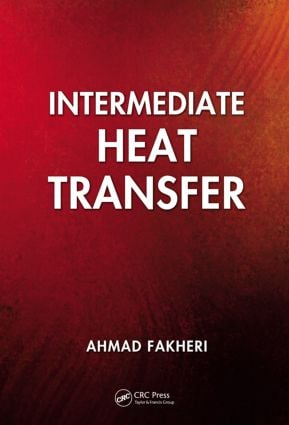# Intermediate Heat Transfer

## 1st Edition

CRC Press

748 pages | 356 B/W Illus.

Paperback: 9780367379810
pub: 2019-07-31
SAVE ~\$14.99
Available for pre-order
\$74.95
\$59.96
x
Hardback: 9781439819364
pub: 2013-07-12
SAVE ~\$35.00
\$175.00
\$140.00
x

FREE Standard Shipping!

### Description

Written for an advanced undergraduate or first-year graduate course, Intermediate Heat Transfer starts with the basics, and puts emphasis on formulating problems, obtaining solutions, and analyzing results using analytical, and numerical methods with the aid of spreadsheets and CFD software. The text employs nondimensionalization as a tool for simplifying the governing equations, developing additional insights into the physics of the problems, identifying the relevant parameters, and arriving at general solutions. It provides comprehensive coverage of the topics and develops the skills for solving heat transfer problems using numerical methods with the aid of spreadsheets and computational fluid mechanics software.

• Presents coverage of convective, conductive, and radiative heat transfer at the graduate level
• Provides a balance of analytical and numerical approaches to advanced heat transfer
• Stresses nondimensionalization throughout the book as a tool for simplifying the governing equations

The author presents detailed numerical solutions to many advanced problems using spreadsheets, although the methods presented for obtaining solutions can be can also be implemented using equation solvers and computing environments, or direct programming using languages such as Fortran or C. The text contains a chapter on CFD to provide the necessary background for obtaining and analyzing CFD solutions. It includes a number of step-by-step tutorials for solving more complicated problems using Fluent, both to show how CFD codes are used as well as a further check of some of the more commonly used assumptions. The text also has extensive coverage of heat exchangers, including being the first text to cover the heat exchanger efficiency for the design and analysis of heat exchangers. This approach eliminates the need for complicated charts or equations. The chapter on mass transfer and chemically reactive flows provides the background needed for modeling of combustion problems.

This book fills the gap between the undergraduate heat transfer course and specialized advanced courses like conduction, convection, radiation, and mass transfer. Much has changed in the field of heat transfer, in what is taught, and how it is presented. An important change has been a shift away from advanced analytical techniques, to more reliance on numerical solutions, which also broadens the topics that are covered in these courses.

### Reviews

"This book…It covers all essential topics in heat transfer."

—Heat Transfer Engineering, Vol. 36 No. 5, 2015

Basic Concepts

Introduction to Heat Transfer

Conservation of Energy

Conduction

Convection

General Heat Diffusion Equation

Coordinate Systems

Initial and Boundary Conditions

One-Dimensional Conduction without Energy Generation

Composite Systems

Contact Resistance

Steady One-Dimensional Conduction with Heat Generation

Closure

Problems

References

Basic Means

Nondimensionalization

Analytical Solutions

Numerical Methods

Problems

References

Fins

Introduction

Quasi One-Dimensional Heat Transfer

Energy Equation

Nondimensionalization

Constant-Area Fins

Variable-Area Fins, Analytical Solutions

Numerical Solution

Fin Arrays

Foam Fins

Problems

References

Multidimensional Conduction

Superposition

Conduction Shape Factors

Numerical Solution

Problems

References

Transient Conduction

Introduction

Lumped Heat Capacity System (Lumped Parameter Method)

Multidimensional System

Numerical Solution of Transient Conduction

Transient Fins

Moving Boundary Problem

Problems

Reference

Convection

Introduction

Conservation Equations

Conservation of Momentum

Conservation of Energy Equation

Index Notation

Streamlines and Stream Function

Nondimensionalization

Problems

Reference

External Flow

Boundary Layer Flows

Boundary Layer Flow over a Flat Plate

Boundary Layer Equations in Curvalinear Coordinates

Boundary Layer over a Horizontal Flat Plate

Nonsimilar Boundary Layer Flow

Jet Flow

Flow over Rotating Surfaces

Problems

References

Internal Flow

Introduction

Couette Flow

General Considerations in Duct Flow

Mean Temperature Analysis

Nusselt Number Calculation

Laminar Fully Developed Flow between Two Parallel Plates (Hagen–Poiseuille Flow)

Laminar Fully Developed Flow in Circular Duct

Hydrodynamically Developed and Thermally Developing Flow

Hydrodynamically and Thermally Developed Flow in Rectangular Duct

Helicoidal Pipes

Moving Solids

Problems

References

Turbulent Flow

Introduction

Solution of Turbulent Flows

Turbulence Models

Turbulent Fully Developed Duct Flow

Turbulent Boundary Layer

Other Turbulence Models

Problems

References

Heat Transfer by Natural Convection

Introduction

Natural Convection Boundary Layer Flow

Combined Natural and Forced Convection

Problems

References

Heat Exchangers

Heat Exchanger Analysis

Heat Exchanger Efficiency

Log Mean Temperature Difference Approach

Effectiveness NTU Method

Heat Exchanger Networks

Compact Heat Exchangers

Microchannel Heat Exchanger

Problems

References

Introduction

Definitions

Blackbody

Problems

References

Participating Medium

Introduction

Energy Balance and Equation of Transfer

Mixed-Mode Heat Transfer

Problems

References

Phase Change

Introduction

Condensation

Film Condensation

Dropwise Condensation

Pool Boiling

Problems

References

Mass Transfer and Reactive Flows

Introduction

Definitions

Law of Conservation of Species

Chemically Reactive Flows

Boundary Layer Flow Transformation

Homogeneous Combustion

Catalytic Combustion

Problems

References

Computational Fluid Dynamics

Introduction

Governing Equations

Spatial Discretization or Grid Generation

Pressure Velocity Coupling

Under-Relaxation

Boundary Conditions

Grid Independence

Tutorial : The Laminar Boundary Layer Flow over a Flat Plate

Tutorial : Turbulent Pipe Flow

Concluding Remarks

Problems

References

Appendix

Index

Peoria, IL, USA